### File information

This PDF 1.4 document has been generated by TeX output 2014.11.17:1617 / MiKTeX-dvipdfmx (20120420), and has been sent on pdf-archive.com on 17/11/2014 at 15:25, from IP address 84.229.x.x. The current document download page has been viewed 754 times.
File size: 69.51 KB (7 pages).
Privacy: public file

### Document preview

Appendix, Paper #140
Adversarial Modeling in the Robotic Coverage
Problem
This document contains the full proofs of the theorems and lemmas that
appear in Paper no. 140, submitted to AAMAS 2015 conference.
Theorem 1. The ZKACP problem is N P-Hard.
Proof. To prove the N P-hardness of the problem, we will use a reduction from
the Hamiltonian path problem, which is known to be N P-complete .
Given an instance of the Hamiltonian path problem on a graph G = (V, E),
we construct an instance of the zero-knowledge adversarial coverage problem on
the same graph G with k = 1. We will prove that there exists a Hamiltonian
path in G, if and only if the optimal strategy for the adversary is to place its
single guard at a random vertex of G.
First direction - if there exists a Hamiltonian path in G, then there is a
coverage path of G that visits each vertex exactly once, which is also the optimal
coverage path. In this case, the best the adversary can do is just place its single
threat randomly in one of the vertices.
Second direction - if G is non-Hamiltonian, then the optimal coverage path
of G must visit one of its vertices more than once. In this case, the optimal
strategy for the adversary is to place its single guard at one of the vertices that
must be visited the greatest number of times. The set of vertices that must be
visited the greatest number of times S is a proper subset of V . This can be easily
veriﬁed by noticing that there are always some vertices in the graph that are
visited only once by the optimal coverage path. For example, the last vertex of
the coverage path must be visited only once, otherwise, we could have removed
its last occurrence from the coverage path and obtain a shorter coverage path
than the optimal one. Additionally, all the leaf vertices in G (those with degree
1) must be visited only once by the optimal coverage path. Thus, the optimal
strategy for the adversary in this case is to place its single guard at one of the
vertices in S, which is diﬀerent from choosing a random vertex of V .
Therefore, we can ﬁnd if there exists a Hamiltonian path in a given graph G,
by checking if the optimal strategy for a zero-knowledge adversary is to place
a single guard randomly in one of the vertices of G. Thus, the zero-knowledge
adversarial coverage problem is N P-hard.

1

Theorem 2. Any coverage path that returns to its starting vertex must visit
every k-connected articulation point at least k times. In addition, if the starting
vertex of the coverage path, s, is a k-connected articulation point then it must
be visited at least k + 1 times.
Proof. Consider a k-connected articulation point v. Removing v from the graph
breaks it into k connected components C1 , ..., Ck . The robot must visit each
of these connected components along its coverage path, and in order to move
between these connected components it must go through v. Assume without
loss of generality that the order of visit of these components is C1 , ..., Ck (the
same component may appear more than once in the sequence). Consider two
cases:
Case 1. v ̸= s. In this case s ∈ C1 . Thus, the coverage path has the following
structure: p = C1
v
C2
v
...
Ck
v
C1 . Hence, the coverage
path must go through v at least k times.
Case 2. v = s. In this case s does not belong to any of the connected
components Ci . Thus, the coverage path has the following structure: p = s
C1
s
...
Ck
s. Hence, the coverage path must visit s at least k + 1
times.
Lemma 1. (correctness) Algorithm 2 computes correctly the connectivity of
each articulation point.
Proof. If the root has more than one child then it is an articulation point,
and the number of connected components its removal splits the graph into is
equal to the number of its child nodes (line 15 in the algorithm). Any other
vertex v is as an articulation point if and only if v has some child w such
that Low(w) ≥ DfsNum(v), i.e., there is a child w of v that cannot reach a
vertex visited before v (line 18). The ﬁrst time this condition is true for v,
it is added to the articulation points list and its connectivity is set to 2 (line
22), since removing v from the graph would split it into at least two connected
components: one that contains the vertices visited before v and another that
contains w and its descendants in the DFS tree. In each subsequent time this
condition becomes true for v, it means that we have found another child w′ of v,
such that v separates w′ from the vertices visited before v, thus removing v from
the graph would create another connected component in the graph (the subtree
rooted in w′ ). Hence, the connectivity of v is increased by 1 (line 24).
Lemma 2. Denote the degree of a vertex v by deg(v). An optimal coverage
path of a graph G = (V, E) that returns to its starting vertex visits every vertex
v ∈ V at most deg(v) times, except for the starting vertex s that is visited at
most deg(s) + 1 times.
Proof. We prove by contradiction. Let Popt be an optimal coverage path of G
that returns to its starting vertex. Assume that there is a vertex v ̸= s in the
graph that is visited by Popt more than deg(v) times. We will show that it is
possible to build a coverage path P ′ that is shorter than Popt .

2

The vertex v is visited at least m = deg(v) + 1 times along Popt . Thus, Popt
has the following structure: Popt = s p1 v p2 v ... v pm v pm+1 s.
Let us denote the last edge on the subpath p1 by e = (u, v). Since v is
connected to only m − 1 diﬀerent edges in G, at least one of the subpaths
p2 , ..., pm must start with the edge e = (u, v). Let us denote the ﬁrst of such
subpaths by pi (2 ≤ i ≤ m). Now, p1 can terminate at vertex u instead of v,
followed by subpath pi that can start at vertex u instead of v, i.e., we can build
the following coverage path: P ′ = s p1 u pi v p2 v ... v pi−1 v pi+1
v ... v pm+1 s. The path P ′ visits all vertices in the graph, but |P ′ | &lt; |Popt |,
since it visits v two times less than Popt . This contradicts the optimality of Popt .
Now, assume that s is visited by Popt more than deg(s) + 1 times, i.e., it is
visited at least m = deg(s) + 2 times. Thus, Popt has the following structure:
Popt = s p1 s p2 s ... s pm−1 s. Let us denote the last edge on the subpath
p1 by e = (u, s). Since s is connected to only m − 2 diﬀerent edges in G, at
least one of the subpaths p2 , ..., pm−1 must start with the edge e = (u, s). Let
us denote the ﬁrst of such subpaths by pi (2 ≤ i ≤ m − 1). Thus, we can build
the following coverage path: P ′ = s p1 u pi s p2 s ... v pi−1 v pi+1
s ... s pm−1 s. The path P ′ visits all vertices in the graph, but |P ′ | &lt; |Popt |,
since it visits s two times less than Popt . This contradicts the optimality of
Popt .
Theorem 3. Let the maximum degree in a graph G be d. If the number of
articulation points in G whose connectivity is d is equal or greater than the
number of guards k, then the adversarial strategy described in algorithm 3 is
optimal.
Proof. By lemma 2, an optimal coverage path that returns to its starting vertex
visits every vertex v ∈ V at most d times, or d+1 times if v is the starting vertex.
By theorem 2, any such coverage path must visit every d-connected articulation
point at least d times, or d + 1 times if it is the starting vertex. Thus, the
optimal coverage path visits every d-connected articulation point precisely d
times if it not the starting vertex, or d + 1 times if it is the starting vertex.
Hence, d-connected articulation points are the most frequently visited vertices
along the optimal coverage path. As a consequence, placing all the given k
guards at these articulation points is guaranteed to maximize the probability to
stop a robot that follows this path.
Theorem 4. The strategy for placing k guards as given by algorithm 3 is not
aﬀected by changing the starting vertex of the coverage, except for maybe a
placement of one guard.
Proof. The block decomposition of a graph is unique. Thus, the number of
articulation points in the graph and their connectivity are not aﬀected by the
selection of the starting vertex of the coverage. According to algorithm 3, if the
starting vertex is an articulation point, then it gets precedence over the other
articulation points with the same connectivity, since it must be visited once
more. Therefore, changing the starting vertex of the coverage may aﬀect the
placement of only the guard at the starting vertex.
3

Theorem 5. Any coverage path must visit every k-connected articulation point
at least k times, except for maybe articulation points on the terminating subpath
of the coverage path, which must be visited at least k − 1 times. In addition, if
the starting vertex of the coverage path is a k-connected articulation point, then
it must be visited at least k + 1 times, unless it is part of the terminating subpath
of the coverage, in which case it is visited at least k times.
Proof. Consider a k-connected articulation point v. Removing v from the graph
breaks it into k connected components C1 , ..., Ck . The robot must visit each
of these connected components along its coverage path, and in order to move
between these connected components it must go through v. Assume without
loss of generality that the order of their visit is C1 , ..., Ck (the same component
may appear more than once in the sequence). Denote the starting vertex of the
coverage path by s. Now, consider two cases:
Case 1. v ̸= s. In this case s ∈ C1 . Thus, the coverage path must have the
following structure: p = C1
v
C2
v
...
Ck . Hence, the coverage
path must go through v at least k − 1 times.
Case 2. v = s. In this case s does not belong to any of the connected
components Ci . Thus, the coverage path must have the following structure:
p=s
C1
s
...
Ck . Hence, the coverage path must visit s at least k
times.
A k-connected articulation point is visited only k − 1 times (or k times if
it is the starting vertex), only if each of its connected components Ci is visited
only once. We now prove that all these articulation points reside on a simple
path ending at the terminating vertex of the coverage path t. Let us denote
these articulation points by v1 , ..., vm , arranged according to the order of their
last visit on the coverage path. vi must belong to the last visited component of
all its previous articulation points vj , j &lt; i, otherwise vj would have to appear
again on the coverage path after vi , violating the ordering v1 , ..., vm .
Now, examine the block tree of G rooted at the starting vertex of the coverage
path s. We show that v1 , ..., vm must belong to the same branch of the block
tree, where each vertex vi is an ancestor of all its successors in the sequence
vi+1 , ..., vm .
We ﬁrst prove that all the vertices vi belong to the same branch by contradiction. Assume that two vertices vi , vj belong to diﬀerent branches of the
block tree. Denote their lowest common ancestor by v. In order to reach vj
from vi , the coverage path must go through v. Thus, the last visited component
Ck of vi contains v. There is a path from v to the root of the block tree s that
does not go through vi , thus v belongs to the same connected component as s,
C1 . Thus, C1 is visited twice, which contradicts the fact that vi is visited only
k − 1 times.
We now prove that vi is an ancestor of vj for every j &gt; i by contradiction.
Assume that vj is an ancestor of vi . vj belongs to the last visited component
of vi . There is a path connecting vj to s which does not go through vi , thus vj
belongs to the same connected component as s, C1 . Thus, C1 is visited twice,
which contradicts the fact that vi is visited only k − 1 times.
4

Since v1 , ..., vm belong to the same branch of the block tree, there is a simple
path in the graph connecting them. Denote this simple path by p. In addition,
the terminating vertex of the coverage path t belongs to the last visited component of vm , Ck , thus vm is an ancestor of t in the block tree. Ck does not
contain any of the vertices v1 , ..., vm , thus there is a simple path p′ from vm
to t that does not go through v1 , ..., vm . Connecting p′ to p generates a simple
path going through v1 , ..., vm and ending at t. Therefore, all the k-connected
articulation points that are visited only k − 1 times belong to the terminating
subpath of the coverage.
Lemma 3. The runtime complexity of algorithm 4 on a graph G = (V, E) is
O(|V |2 ).
Proof. In the worst case scenario, the graph G consists of a linear chain of nodes,
since in this case all the vertices in the graph, except for two, are articulation
points and the articulation points tree T contains only one branch. Thus, the
procedure Place Guards is invoked once for each articulation point, each time
placing only one guard. This procedure scans T until reaching a vertex whose
all descendants contain guards, thus the number of vertices it visits in each
scan decreases by one. Hence, the total number of visits to vertices in T is
n + (n − 1) + ... + 1 = O(n2 ), where n is the number of vertices in T . Since
n = O(V ), the total running time of the algorithm is O(|V |2 ).
Theorem 6. Let U be a k-connected vertex cut. Then any coverage path that
returns to its starting vertex must visit vertices in U at least k times. In addition,
if the starting vertex of the coverage path belongs to U , then the vertices in U
must be visited at least k + 1 times.
Proof. Consider a k-connected vertex cut U . Removing U from the graph breaks
it into k connected components C1 , ..., Ck . The robot must visit each of these
connected components along its coverage path, and in order to move between
these connected components it must go through one of the vertices in U . Assume
without loss of generality that the order of visit of these components is C1 , ..., Ck
(the same component may appear more than once in the sequence). Denote the
starting vertex of the coverage path by s. Now, consider two cases:
Case 1. s ̸∈ U . In this case s ∈ C1 . Thus, the coverage path must have the
following structure: p = C1
U
C2
U
...
Ck
U
C1 . Hence,
the coverage path must go through vertices in U at least k times.
Case 2. s ∈ U . In this case s does not belong to any of the connected
components Ci . Thus, the coverage path must have the following structure:
p=s
C1
U
C2
U
...
Ck
s. Hence, the coverage path must
go through vertices in U at least k + 1 times (two of these visits belong to the
starting vertex).
Theorem 7. Let U be a k-connected vertex cut. Then any coverage path must
visit vertices in U at least k − 1 times. In addition, if the starting vertex of
the coverage path belongs to U , then the vertices in U must be visited at least k
times.
5

Proof. Consider a k-connected vertex cut U . Removing U from the graph breaks
it into k connected components C1 , ..., Ck . The robot must visit each of these
connected components along its coverage path, and in order to move between
these connected components it must go through one of the vertices in U . Assume
without loss of generality that the order of visit of these components is C1 , ..., Ck
(the same component may appear more than once in the sequence). Denote the
starting vertex of the coverage path by s. Now, consider two cases:
Case 1. s ̸∈ U . In this case s ∈ C1 . Thus, the coverage path must have the
following structure: p = C1
U
C2
U
...
Ck . Hence, the coverage
path must go through vertices in U at least k − 1 times.
Case 2. s ∈ U . In this case s does not belong to any of the connected
components Ci . Thus, the coverage path must have the following structure:
p=s
C1
U
C2
U
...
Ck . Hence, the coverage path must go
through vertices in U at least k times.
Lemma 4. Any vertex cut that does not contain a proper subset which is also
a vertex cut, belongs entirely to one biconnected component.
Proof. Consider a vertex cut C. Assume by contradiction that is does not belong
entirely to one biconnected component, i.e., its vertices belong to two or more
blocks in the graph. Denote these blocks by B1 , ..., Bn and the subsets of vertices
in C that belong to these blocks by V1 , ..., Vn . From the block decomposition of
the graph we know that there is a path that connects all the blocks in the graph
and goes through the articulation points. Since all the vertices in C are not
articulation points, removing C from the graph does not aﬀect the path that
connects B1 , ..., Bn . Thus, removing C must break at least one of the blocks, Bi .
Since the only vertices in C that aﬀect the connectivity of Bi are the vertices
in Vi , the subset Vi must also be a vertex cut. This contradicts the fact that C
does not contain a proper subset which is also a vertex cut.
(( )
)
Lemma 5. The runtime complexity of Find Vertex Cuts is O |Vk | ·(|V |+|E|) ,
where k is the given vertex cut size.
Proof. In the worst case scenario, G is biconnected and there is no node in G
that contains guards (for example, when G does not contain articulation points,
then the ﬁrst time the procedure is called there will be no guards placed in G).
In this case, GB = G and F contains
all the nodes in G. Thus, the number
( )
of subsets of k nodes in F is |Vk | . For each subset, we ﬁnd the connected
components in G without the subset by running DFS, whose running time is
O(|V | + |E|). Hence, in the worst case the total running time of the procedure
(( )
)
is O |Vk | · (|V | + |E|) .
(
)
Lemma 6. The runtime complexity of algorithm 5 is O 2|V | · (|V | + |E|) .
Proof. In the worst case scenario, the graph G is biconnected and all the vertex
cuts of the graph have to be computed. In this case, the algorithm needs to
examine all the subsets of vertices
in V (whose size is greater than 1). The
(
number of these subsets is O 2|V | ). For each subset, the algorithms runs DFS
6

in order to ﬁnd the connected components in G without the subset. Since the
running time of DFS(is O(|V | + |E|), )in the worst case the total running time
of the algorithm is O 2|V | · (|V | + |E|) .

References
 M. R. Garey and D. S. Johnson. Computers and intractability: a guide to
the theory of np-completeness. Freeman, San Francisco, 1979.

7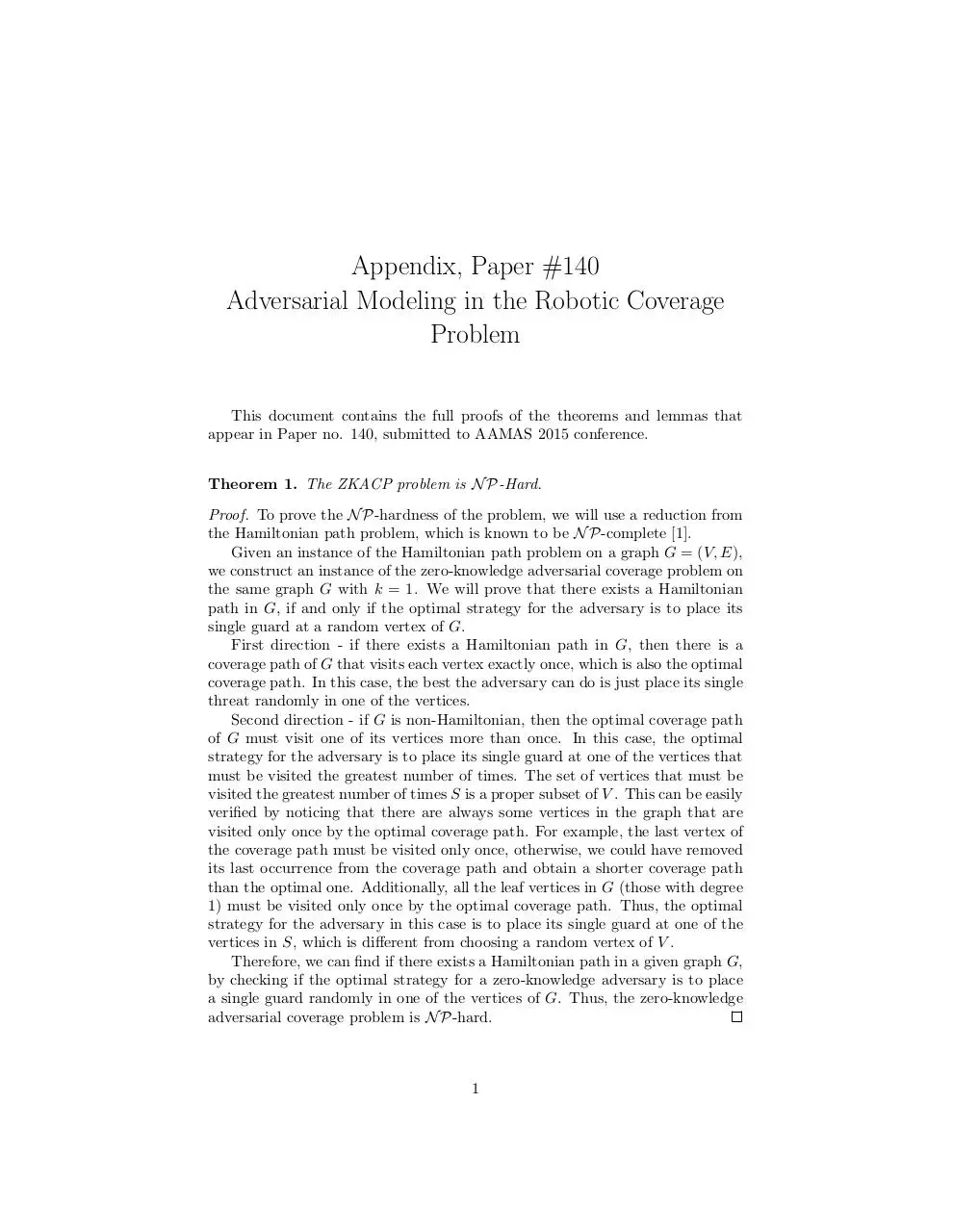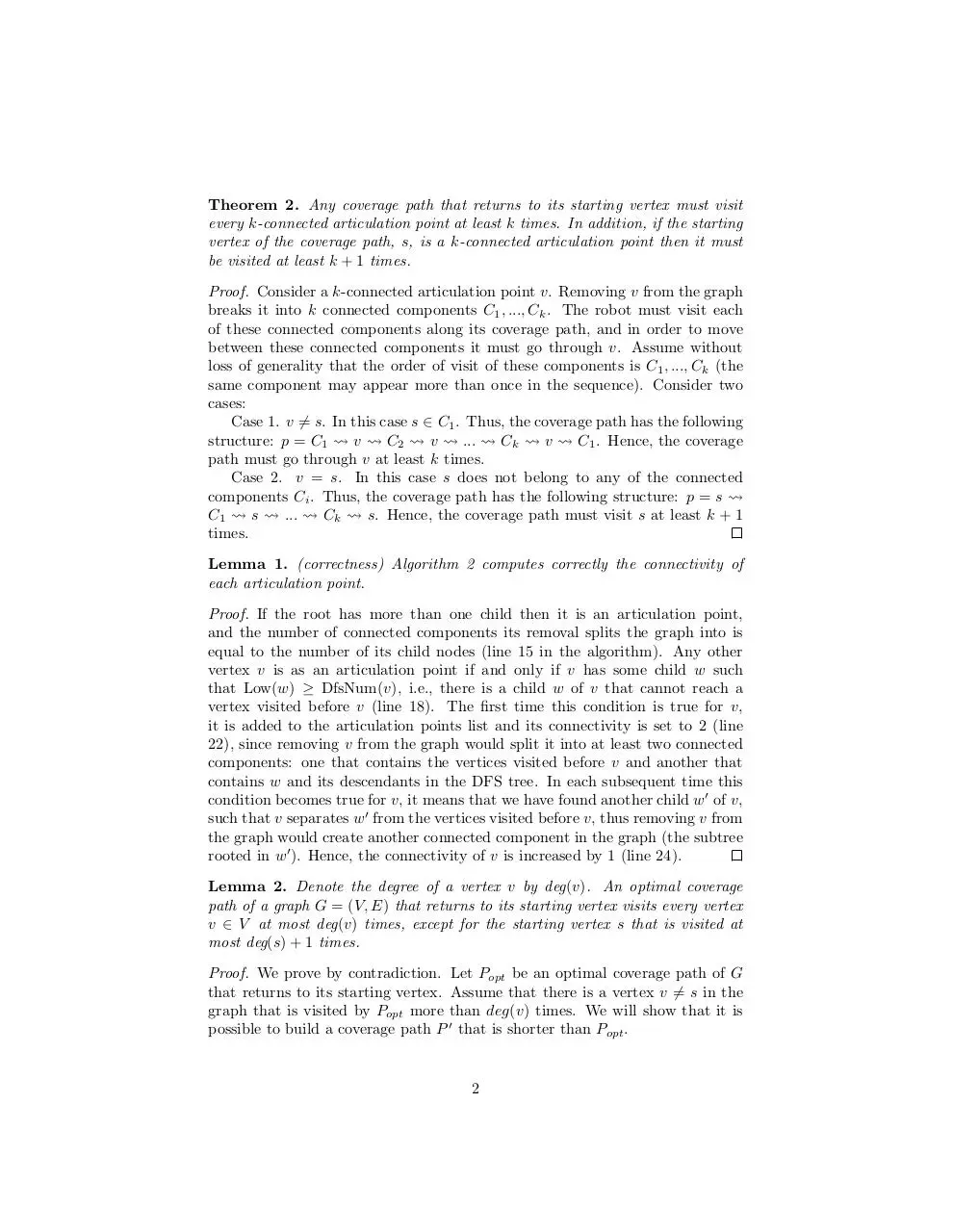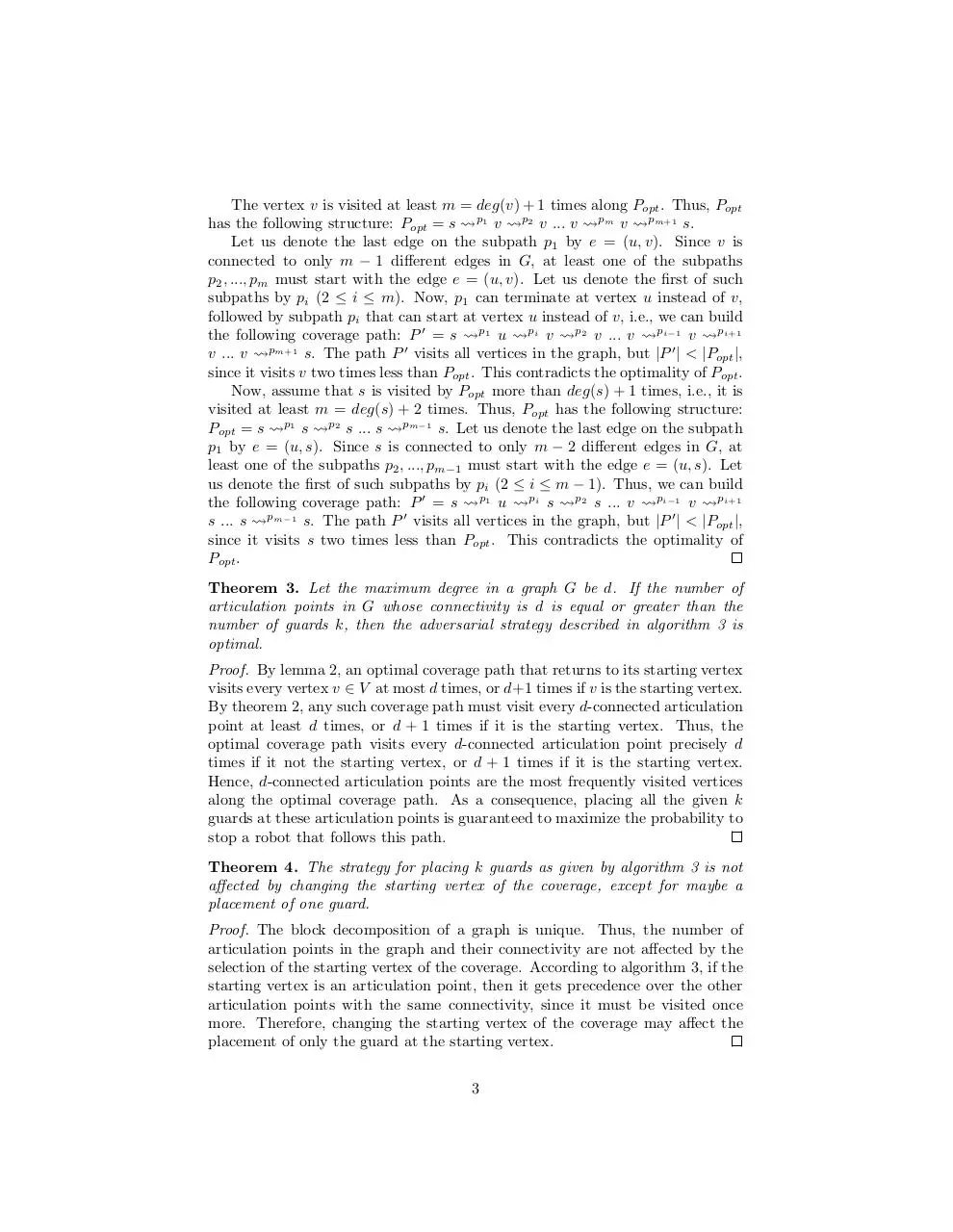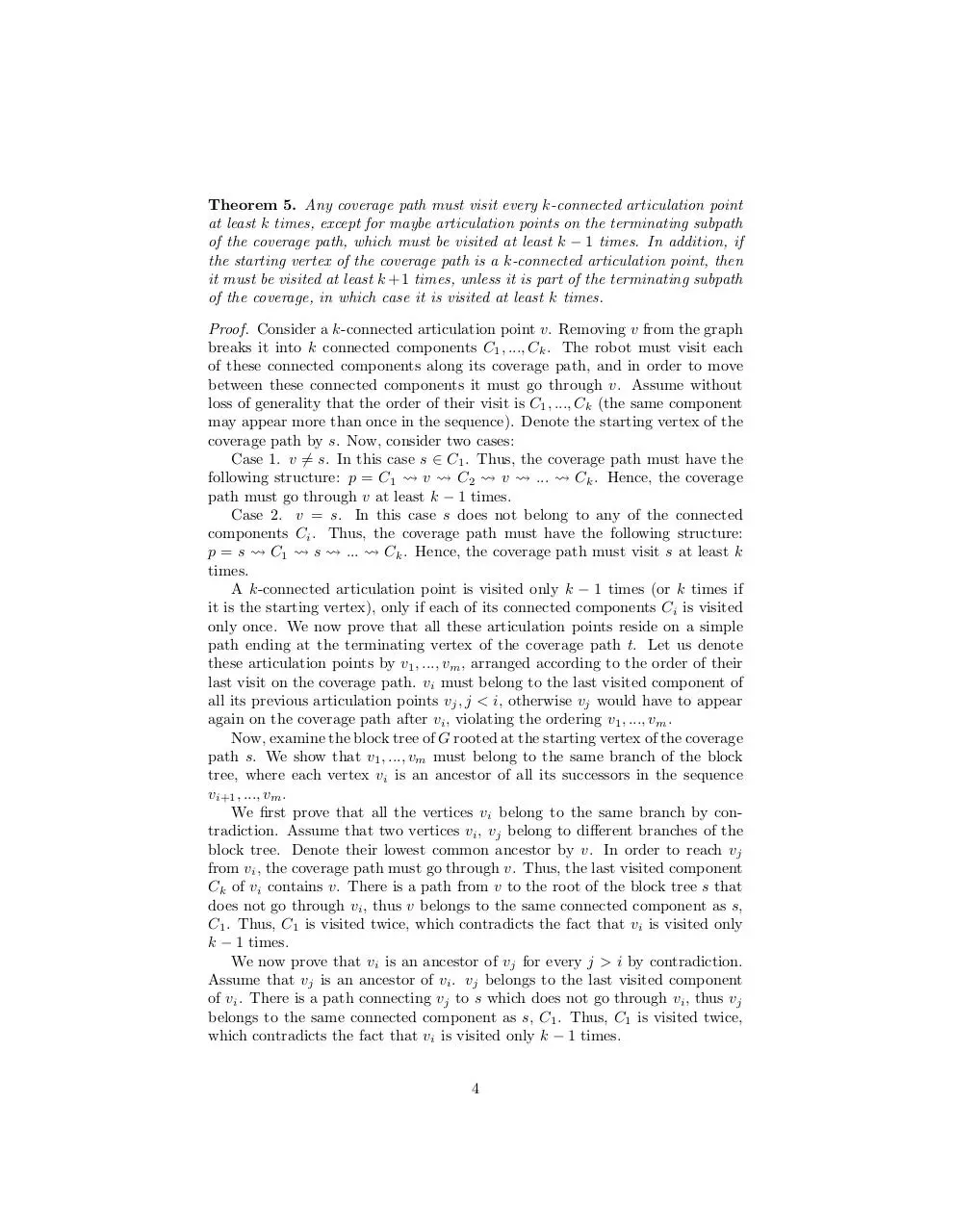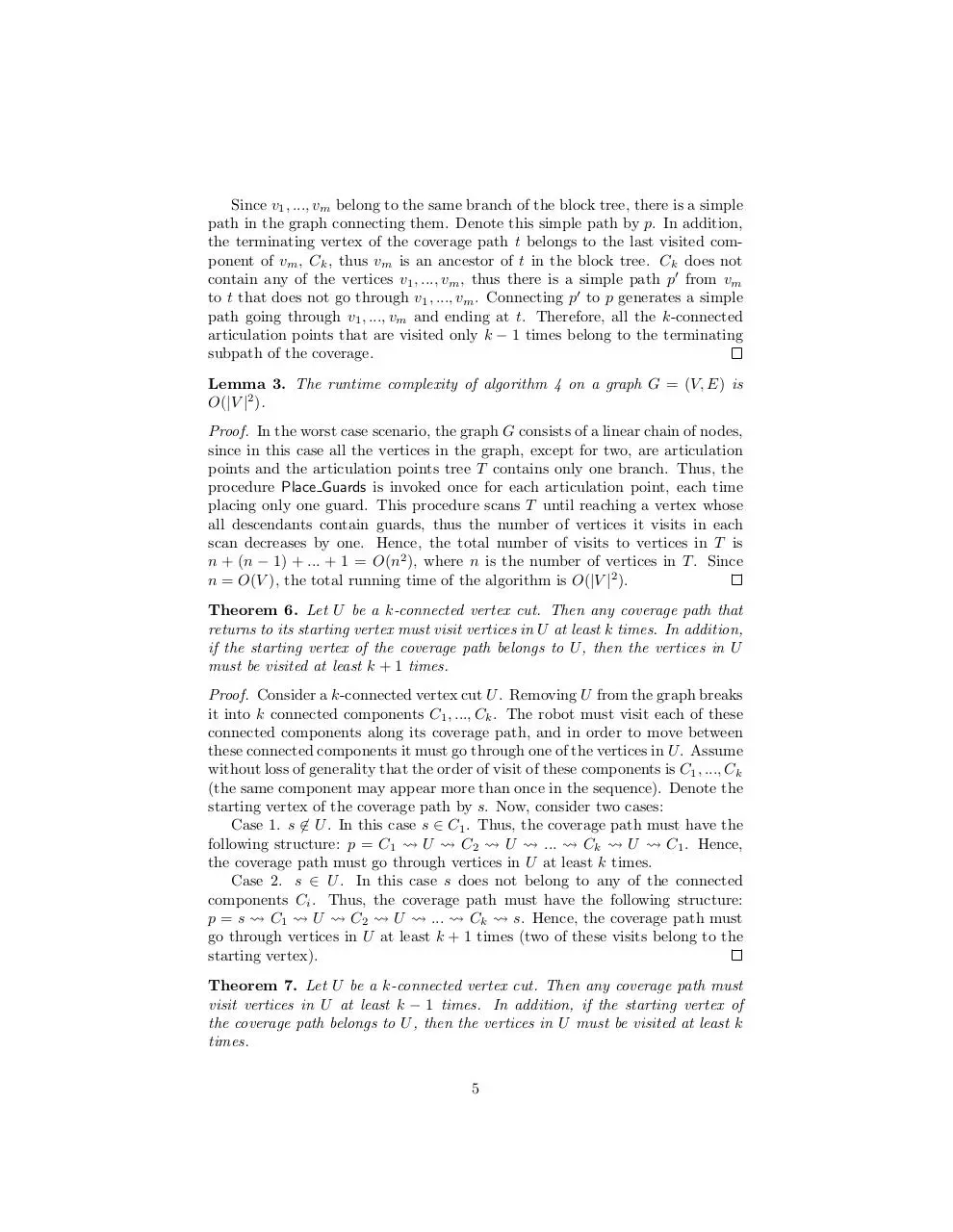### Share on social networks

#### HTML Code

Copy the following HTML code to share your document on a Website or Blog